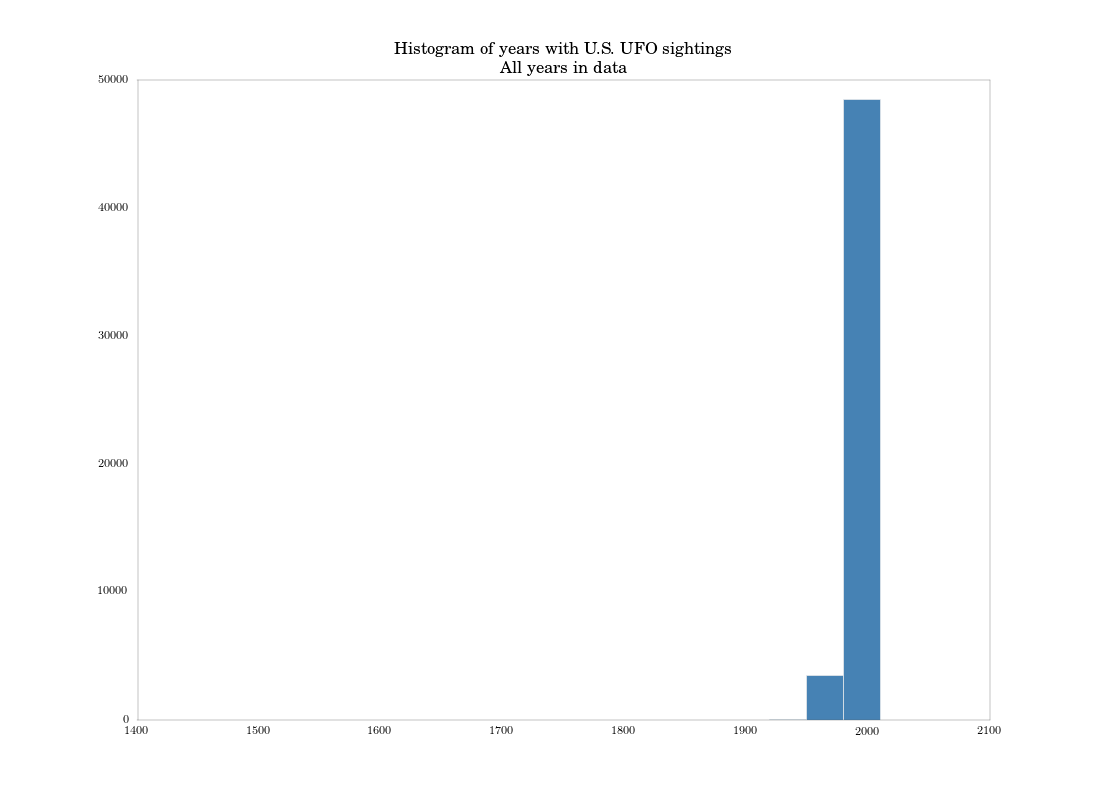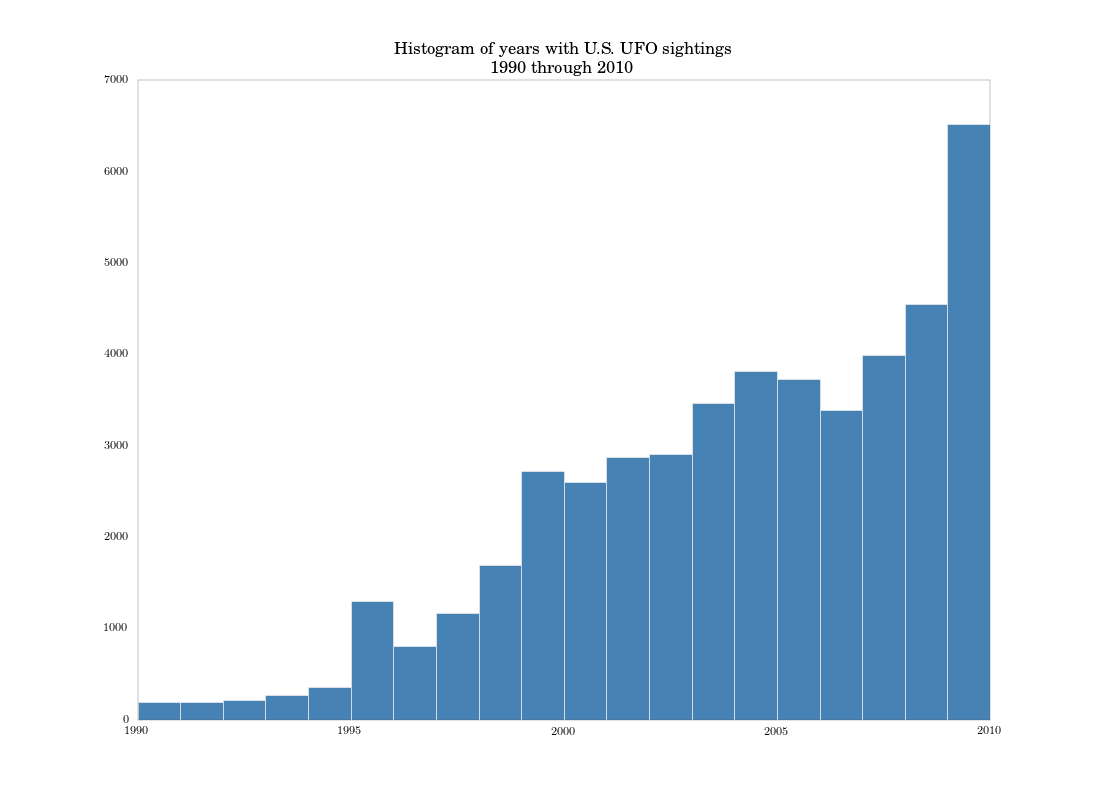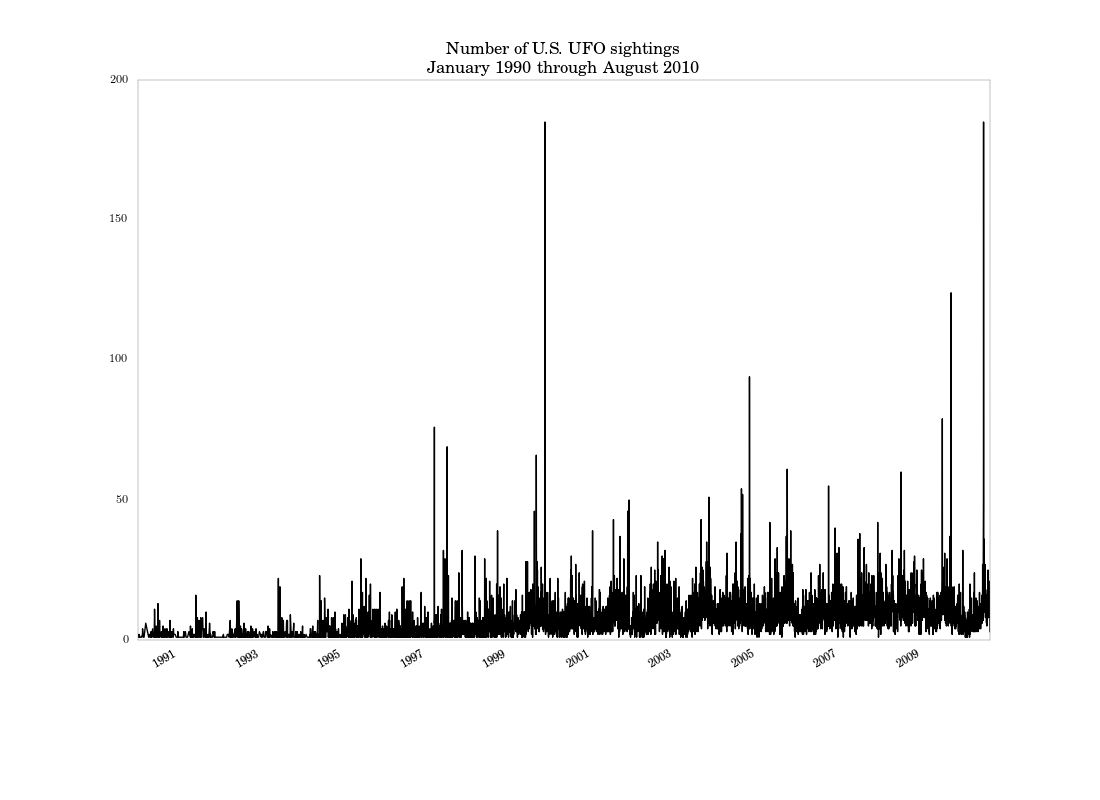# Machine Learning for Hackers Chapter 1, Part 3: Simple summaries and plots.

## Introduction

See Part 1 and Part 2 for previous work.

In this part, I’ll replicate the authors’ exploration of the UFO sighting dates via histograms. The key takeaways:

1. The plotting methods in Pandas are easy and useful.
2. Unlike R `Dates`, Python `datetimes` aren’t compatible with a lot of mathematical operations. We’ll see that you can’t apply quantile or histogram methods to them directly.

## Quick data summary methods and datetime complications.

For those playing along at home, I’m at p. 19 of the book. The first thing the authors do here is get a statistical summary of the sighting dates in the data, which are recorded in the `DateOccurred` variable (which I’ve named `date_occurred` in my code). This is easy in R using the `summary` function, which provides the minimum, maximum, and quartiles of the data by default.

Pandas has similar functionality, in a method called `describe`, which gives the same for numeric variables, plus the count of non-null values and the mean and standard deviation. For example:

```s1 = Series(np.random.randn(100))
print s1.describe()
```

outputs what we’d expect from a series of randomly-generated standard normals:

```count 100.000000
mean -0.149274
std 1.011230
min -2.521374
25% -0.790867
50% -0.167813
75% 0.596617
max 2.231157
```

If we apply this to the `date_occurred` series, though, we get something different.

```ufo_us['date_occurred'].describe()[/sourcecode]
```

results in:

```count 52134
unique 8786
top 1999-11-16 00:00:00
freq 185
```

because Pandas treats `datetime` series as non-numeric variables (which they technically are).

Note: To compute quantiles for numeric series, Pandas uses SciPy’s `scoreatpercentile` function, which in turn relies on a simple linear interpolation function (`_interpolate` in `scipy.stats`). `datetime` objects don’t play well with this function, since when you take the difference between two `datetimes` you don’t get a number, but instead a `timedelta` tuple, that you can’t perform mathematical operations on until you unpack it. The `min` and `max` methods will work on `datetimes`, though.

We can get around this by extracting the years from the variable, which will be integers.

```years = ufo_us['date_occurred'].map(lambda x: x.year)
print years.describe()
```

results in:

```count 52134.000000
mean 2000.572237
std 10.889045
min 1400.000000
25% 1999.000000
50% 2003.000000
75% 2007.000000
max 2010.000000
```

which is a little precise for year data, but how is Pandas to know? At any rate, we come to the same conclusion as the authors: that three quarters of the sightings occurred in 1999 or later, and the earliest date in the data is in 1400. (If we check, we’ll see this sighting occurred in Texas, so it’s certainly an error).

Plotting histograms

The authors then plot a histogram of the dates in the data. Like with `quantile`, the `hist` plot method (which just calls a Matplotlib histogram) doesn’t work with `datetime` data. If we try

```ufo_us['date_occurred'].hist()
```

we’ll get an error complaining that `datetime` can’t be compared with `float`. So, I’ll just work with the years instead of the full `datetime`. I can generate the plot with a call to the series’ `hist` method, one of several plotting methods for Pandas objects that makes it extremely easy to get quick plots of them.

```plt.figure()
years.hist(bins = (years.max() - years.min())/30., fc = 'steelblue')
plt.title('Histogram of years with U.S. UFO sightings\nAll years in data')
plt.savefig('quick_hist_all_years.png')
```

I explicitly set the bins to match the ggplot defaults used in the book. We get this plot, which basically matches the authors’:The authors then focus on only data after 1990, using R’s `subset` function to remove earlier observations from the data. This is straightforward in Pandas. I’ll also extract another series with the years of this subset of dates.

```ufo_us = ufo_us[ufo_us['date_occurred'] \>= dt.datetime(1990, 1, 1)]
years_post90 = ufo_us['date_occurred'].map(lambda x: x.year)
```

After subsetting, the authors have 46,347 rows left in the data. Looking at the `shape` attribute of the subsetted data frame, we have 46,780. We’ve picked up some observations from D.C., as well as from our more expansive method of finding U.S. locations.

Another histogram of the subset data looks similar to the authors’ chart on p. 23, but since I’m only histogramming over years, I lose some resolution.While the histogram is fine for a quick look at the distribution of dates, it’s not a very accurate picture of how sightings evolve over time: the binning really destroys too much information. It makes more sense just to do a time-series plot of total sightings by date. We can do that with some data aggregation and an easy call to the `plot` method in Pandas.

```post90_count = ufo_us.groupby('date_occurred')['date_occurred'].count()
plt.figure()
post90_count.plot()
plt.title('Number of U.S. UFO sightings\\nJanuary 1990 through August 2010')
plt.savefig('post90_count_ts.png')
```

This uses Pandas’ awesome `groupby` method, which I’ll discuss more in the next part. We get the following figure:Based on this graph, it looks like there’s a seasonal component to sightings, which wasn’t apparent in the histogram. There are also a few large spikes, especially around the end of the millenium.

## Conclusion

This part was a relatively easy one. The next part will focus on data aggregation using `groupby` and `reindex` methods. Then I’ll wrap up with with replicating the authors’ trellis graph.